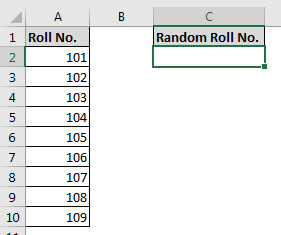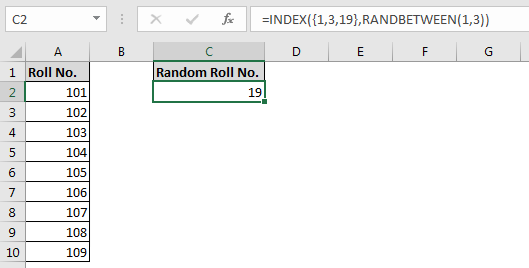# Get Random number From Fixed Options

It is quite easy to select random numbers from a fixed set of numbers using INDEX and RANDBETWEEN function of excel.

Generic Formula

=INDEX(number_list, RANDBETWEEN(1, COUNT(number_list))

Number_List: this is the fixed list from which you want to get random number.
Example: Select Random Roll Number From Given List Only
Here I have a list of number in range A2:A10. Now in cell C2, I want to select a random number from this list of numbers only.Apply above generic formula here to get a random number from fixed list.

=INDEX(A2:A10, RANDBETWEEN(1, COUNT(A2:A10))

This gives a random roll number from the list of roll numbers we specified. Everytime you make a change in sheet this formula recalculates and gives another random number from that list only.How it works?

Well, this quite easy. We all know RANDBETWEEN function returns any number from a given range. If we wanted a random number between 1 and 100 we would write  RANDBETWEEN(1, 100). Here the upper limit is set by COUNT function. Lets break it down.

COUNT(A2:A10): this part returns the count of numbers in range A2:A10 which is 9. Now the formula is simplified to

INDEX(A2:A10, RANDBETWEEN(1, 9)): the randbetween returns any number between range 1 to 9. Let’s say it returns 5. Now the formula is simplified to.

INDEX(A2:A10, 5): this return the 5th number in range A2:A10.
Get Random Number From Hardcoded Numbers
If you want to get random number from a fixed list that is not going to change, then consider using below formula.

=INDEX({1,5,10,12}, RANDBETWEEN(1, 4))

The CHOOSE Alternative
If you have a fixed list as above then this CHOOSE formula will also get you a random number from specified list.

=CHOOSE( RANDBETWEEN(1, 4),1,5,10,12)

So yeah guys, this how you can return a random number from a fixed number list. Let me know if you have any other techniques. If you have any doubts regarding this article or any other excel topic, let me know in the comments section below.

Related Articles:

Get Random numbers between two numbers.

Generate Random Phone Numbers

Excel Random Selection: How to Get Random Sample From a Dataset

Popular Articles:

The VLOOKUP Function in Excel

COUNTIF in Excel 2016

How to Use SUMIF Function in Excel

Terms and Conditions of use

The applications/code on this site are distributed as is and without warranties or liability. In no event shall the owner of the copyrights, or the authors of the applications/code be liable for any loss of profit, any problems or any damage resulting from the use or evaluation of the applications/code.